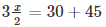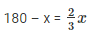Courses

# RD Sharma Solutions Ex-8.1, Lines And Angles, Class 9, Maths Class 9 Notes | EduRev

## Class 9 : RD Sharma Solutions Ex-8.1, Lines And Angles, Class 9, Maths Class 9 Notes | EduRev

The document RD Sharma Solutions Ex-8.1, Lines And Angles, Class 9, Maths Class 9 Notes | EduRev is a part of the Class 9 Course RD Sharma Solutions for Class 9 Mathematics.
All you need of Class 9 at this link: Class 9

Q 1 : Write the complement of each of the following angles:

(i)20
(ii)35
(iii)90
(iv)77
(v)30

Ans : (i) given angle is 20

Since, the sum of an angle and its compliment is 90

Hence, its compliment will be (90 – 20 = 70)

(ii) Given angle is 35

Since, the sum of an angle and its compliment is 90

Hence, its compliment will be (90 – 35 = 55)

(iii) Given angle is 90

Since, the sum of an angle and its compliment is 90

Hence, its compliment will be (90 – 90 = 0)

(iv) Given angle is 77

Since, the sum of an angle and its compliment is 90

Hence, its compliment will be (90 – 77 = 13)

(v) Given angle is 30

Since, the sum of an angle and its compliment is 90

Hence, its compliment will be (90 – 30 = 60)

Q 2 : Write the supplement of each of the following angles:

(i) 54
(ii) 132
(iii) 138

Ans : (i) The given angle is 54,

Since the sum of an angle and its supplement is 180,

Hence, Its supplement will be (180 – 54 = 126)

(ii) The given angle is 132,

Since the sum of an angle and its supplement is 180,

Hence, its supplement will be 180 – 132 = 48

(iii) The given angle is 138,

Since the sum of an angle and its supplement is 180,

Hence, Its supplement will be 180 – 138  = 42

Q 3 : If an angle is 28 less than its complement, find its measure?

Ans: Let the angle measured be ‘ x ‘ in degrees

Hence, Its complement will be 90−x

• Angle = Complement – 28
• x = (90 – x) – 28
• 2x = 62
• x = 31

Therefore, angle measured is 31

Q 4 : If an angle is 30 more than half of its complement, find the measure of the angle?

Ans : Let the measured angle be ‘x‘

Hence its complement will be (90-x)

It is given that,

Angle =30 + complement/2

• x = 30 + (90 – x) / 2
•• 3x = 150
• x = 50

Therefore the angle is 50

Q 5 : Two supplementary angles are in the ratio 4:5.Find the angles?

Ans : Supplementary angles are in the ratio 4:5

Let the angles be 4x and 5x

It is given that they are supplementary angles

Hence 4x + 5x = 180

• 9x = 180
• x = 20

Hence, 4x = 4 (20) = 80

5(x) = 5 (20) = 100

Hence, angles are 80 and 100

Q 6 : Two supplementary angles differ by 48∘.Find the angles ?

Ans : Given that two supplementary angles differ by 48

Let the angle measured be x∘

Therefore, Its supplementary angle will be (180−x)

It is given that :

• (180 – x) – x = 48
• (180 – 48) = 2x
• 2 x = 132
• x = 132/2
• x = 66

Hence, 180 – x = 114

Therefore, the angles are 66 and 114.

Q 7 : An angle is equal to 8 times its complement. Determine its measure?

Ans : It is given that required angle = 8 times its complement

Let ‘ x ‘ be the measured angle

• angle = 8 times complement
• angle = 8 (90 – x)
• x = 8 (90 – x )
• x = 720 – 8x
• x + 8x = 720
• 9x = 720
• x = 80

Therefore measured angle is 80.

Q 8 : If the angles (2x−10) and (x−5) are complementary, find x ?

Ans : Given that (2x−10)∘ and (x−5)∘ are complementary

Since angles are complementary, their sum will be 90

• (2x – 10) + (x – 5) = 90
• 3x -15 = 90
• 3x = 90 + 15
• 3x = 105
• x = 105/3
• x = 35

Hence, the value of x = (35)

Q 9 : If the compliment of an angle is equal to the supplement of Thrice of itself, find the measure of the angle ?

Ans : Let the angle measured be ‘ x ‘ say.

Its complementary angle is (90 – x) and

Its supplementary angle is (180 – 3x)

Given that, Supplementary of 4 times the angle = (180 – 3x)

According to the given information;

• (90 – x) = (180 – 3x)
• 3x – x = 180 – 90
• 2x = 90
• x = 90/2
• x = 45

Therefore, the measured angle x = (45)

Q 10 : If an angle differs from its complement by (10), find the angle ?

Ans : Let the measured angle be ‘ x ‘ say

Given that,

The angles measured will differ by (20)

x – (90 – x) = 10

• x – 90 + x = 10
• 2x = 90 + 10
• 2x = 100
• x = 100/2
• x = 50

Therefore the measure of the angle is (50)

Q 11 : If the supplement of an angle is 3 times its complement, find its angle ?

Ans : Let the angle in case be ‘ x ‘

Given that,

Supplement of an angle = 3 times its complementary angle

Supplementary angle = 180 – x

Complementary angle = 90 – x

Applying given data,

• 180 – x = 3 (90 –x)
• 3x – x = 270 – 180
• 2x = 90
• x = 90/2
• x = 45

Therefore, the angle in case is 45

Q 12 : If the supplement of an angle is two third of itself. Determine the angle and its supplement?

Ans:  Supplementary of an angle = 2/3 angle

Let the angle in case be ‘ x ’,

Supplementary of angle x will be ( 180 – x)

It is given that• (180 – x) 3 = 2x
• 540 -3x = 2x
• 5x = 540
• x = 540/5
• x = 108

Hence, supplementary angle = 180 – 108 = 72

Therefore, angles in case are 108 and supplementary angleis 72

Q 13 : An angle is 14 more than its complementary angle. What is its measure?

Ans:  Let the angle in case be ‘ x ’,

Complementary angle of ‘ x ’ is ( 90 – x)

From given data,

x – (90 – x) = 14

• x – 90 +x = 14
• 2x = 90 + 14
• 2x = 104
• x =104/2
• x = 52

Hence the angle in case is found to be 52∘

Q 14 : The measure of an angle is twice the measure of its supplementary angle. Find the measure of the angles?

Ans : Let the angle in case be ‘ x ’

The supplementary of a angle x is (180 – x)

Applying given data:

x = 2 (180 –x)

• x = 360 -2x
• 3x = 360
• x = 360/3
• x = 120

Therefore the value of the angle in case is 120

Offer running on EduRev: Apply code STAYHOME200 to get INR 200 off on our premium plan EduRev Infinity!

91 docs

,

,

,

,

,

,

,

,

,

,

,

,

,

,

,

,

,

,

,

,

,

,

,

,

,

,

,

,

,

,

;